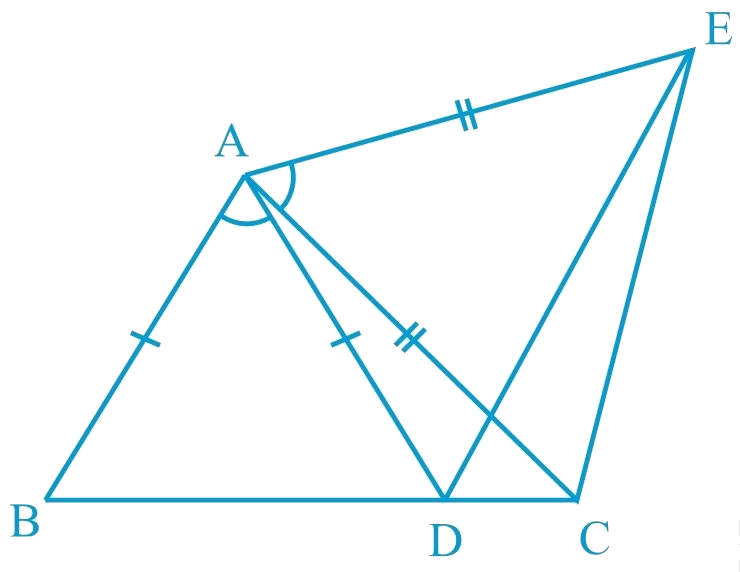In the verge of coronavirus pandemic, we are providing FREE access to our entire Online Curriculum to ensure Learning Doesn't STOP!

# Ex.7.1 Q6 Triangles Solution - NCERT Maths Class 9

Go back to  'Ex.7.1'

## Question

In the given figure, $$AC = AE$$, $$AB = AD$$

and $$\angle BAD = \angle EAC$$.

Show that $$BC = DE$$.

Video Solution
Triangles
Ex 7.1 | Question 6

## Text Solution

What is Known?

$$AC = AE, AB = AD$$ and

$$\angle BAD = \angle EAC.$$

To prove:

$$BC = DE.$$

Reasoning:

We can show two triangles BAC and DAE congruent by using SAS congruency rule and then we can say corresponding parts of congruent triangles will be equal. To show both triangles congruent two pair of equal sides are given and add angle DAC on both sides in given pair of angles BAD and angle EAC to find the included angle BAC and DAE.Steps:

It is given that

$$\angle BAD = \angle EAC$$

$$\angle BAD\!+\!\angle DAC\!=\!\angle EAC\!+\!\angle DAC$$

$$\angle BAC = \angle DAE$$

In $$\Delta BAC$$ and $$\Delta DAE$$,

\begin{align} AB & =AD\,(\text{Given})\, \\ \angle BAC&=\angle DAE\, \\ & (\text{Proven}\,\,\text{above})\, \\ & \\ AC&=AE\,(\text{Given})\, \\ \therefore \Delta BAC&\cong \Delta DAE\, \\ (\text{By}\,\text{SAS cong}&\text{ruencerule}) \\ & \\\therefore BC & =DE\,(ByCPCT) \\ \end{align}

Video Solution
Triangles
Ex 7.1 | Question 6

Learn from the best math teachers and top your exams

• Live one on one classroom and doubt clearing
• Practice worksheets in and after class for conceptual clarity
• Personalized curriculum to keep up with school